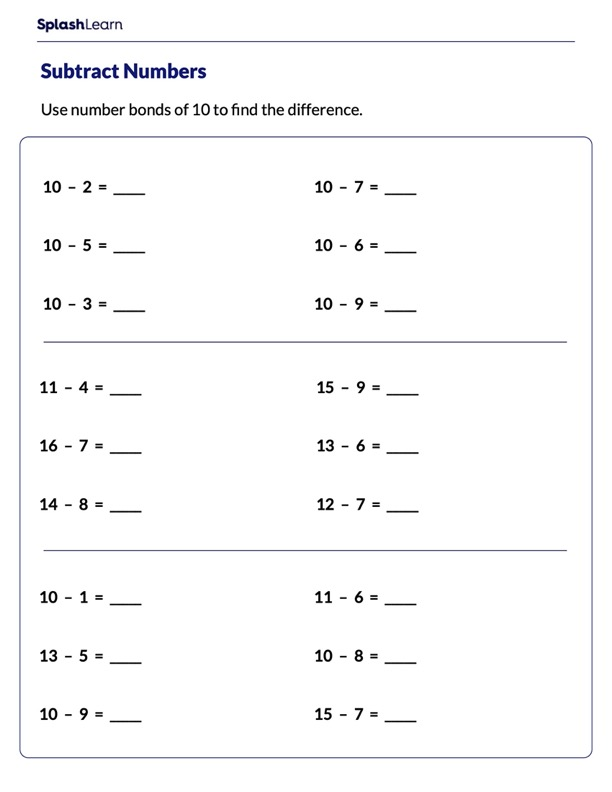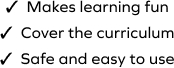# Subtract Using Number Bonds of 10

SplashLearn - The complete PreK - Grade 5 Math & ELA Learning Program Built for Your Child
Home > Subtract Using Number Bonds of 10Building a strong foundation in subtraction is an important step in helping your child become proficient and confident. The worksheet involves working with a set of problems on subtraction strategies to find the answer, which helps young learners solidify their understanding of the concept. Here students apply the split into tens strategy to make the calculation simpler.